## 版权声明：转载必须注明本文转自程序员杜鹏程的博客:http://blog.csdn.net/m366917

• Set
• 元素是无序(存储顺序和取出顺序不一致),元素是唯一的，不可重复的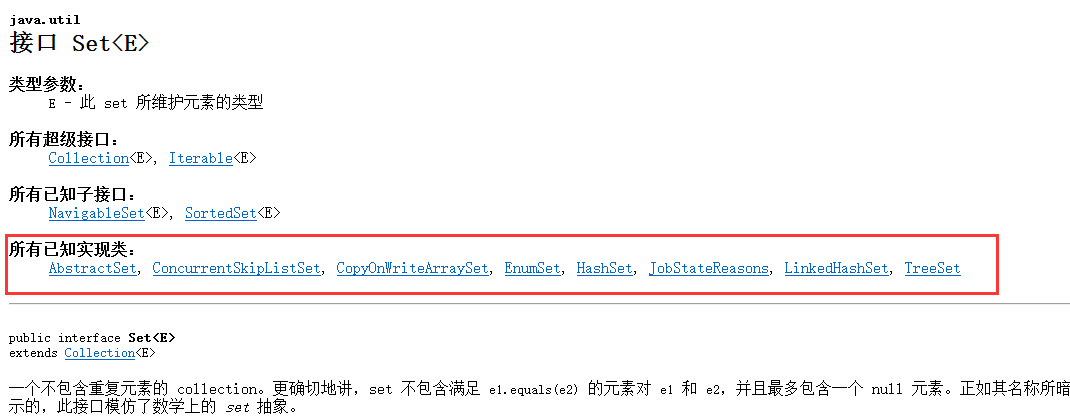public class SetDemo {
public static void main(String[] args) {
// 创建集合对象
Set<String> set = new HashSet<String>();

// 创建并添加元素

// 增强for遍历
for (String s : set) {
System.out.println(s);
}
//迭代器遍历
Iterator it = set.iterator();
while(it.hasNext()){
System.out.println(it.next());
}
}
}

## 增强for

格式：
for(元素数据类型 变量 : 数组或者Collection集合) {
使用变量即可，该变量就是元素
}

## HashSet类

• HashSet类概述
• 不保证 set 的迭代顺序
• 特别是它不保证该顺序恒久不变。
• HashSet如何保证元素唯一性
• 底层数据结构是哈希表(元素是链表的数组)
• 哈希表依赖于哈希值存储
• 添加功能底层依赖两个方法：
• int hashCode()
• boolean equals(Object obj)
public class HashSetDemo {
public static void main(String[] args) {
// 创建集合对象
HashSet<String> hs = new HashSet<String>();

// 创建并添加元素

// 遍历集合(这里可以用增强for也可以用迭代器，但是增强for比较简单点)
for (String s : hs) {
System.out.println(s);
}
}
}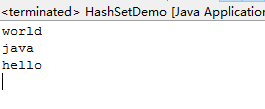• 步骤：
• 首先比较哈希值
• 如果相同，继续走，比较地址值或者走equals()
• 如果不同,就直接添加到集合中
• 按照方法的步骤来说：
• 先看hashCode()值是否相同
• 相同:继续走equals()方法
• 返回true： 说明元素重复，就不添加
• 返回false：说明元素不重复，就添加到集合
• 不同：就直接把元素添加到集合
• 如果类没有重写这两个方法，默认使用的Object()。一般来说不同相同。
• 而String类重写了hashCode()和equals()方法，所以，它就可以把内容相同的字符串去掉。只留下一个。

## 存储自定义对象

public class HashSetDemo {
public static void main(String[] args) {
// 创建集合对象
HashSet<Student> hs = new HashSet<Student>();

// 创建学生对象
Student s1 = new Student("朱婷", 22);
Student s2 = new Student("惠若琪", 22);
Student s3 = new Student("徐云丽", 21);
Student s4 = new Student("朱婷", 22);
Student s5 = new Student("郎平", 55);
Student s6 = new Student("郎平", 57);

// 添加元素

// 遍历集合
for (Student s : hs) {
System.out.println(s.getName() + "---" + s.getAge());
}
}
}

/**
* 存储对象
*/
public class Student {
private String name;
private int age;

public Student() {
super();
}

public Student(String name, int age) {
super();
this.name = name;
this.age = age;
}

public String getName() {
return name;
}

public void setName(String name) {
this.name = name;
}

public int getAge() {
return age;
}

public void setAge(int age) {
this.age = age;
}
}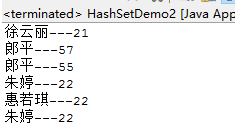@Override
public int hashCode() {
final int prime = 31;
int result = 1;
result = prime * result + age;
result = prime * result + ((name == null) ? 0 : name.hashCode());
return result;
}

@Override
public boolean equals(Object obj) {
if (this == obj)
return true;
if (obj == null)
return false;
if (getClass() != obj.getClass())
return false;
Student other = (Student) obj;
if (age != other.age)
return false;
if (name == null) {
if (other.name != null)
return false;
} else if (!name.equals(other.name))
return false;
return true;
}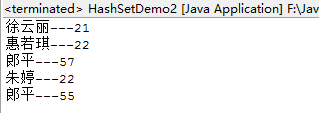• 概述
• 底层数据结构由哈希表和链表组成
• 由链表保证元素有序(存储和取出是一致)
• 由哈希表保证元素唯一性
我们做个小练习来了解它
public class LinkedHashSetDemo {
public static void main(String[] args) {
// 创建集合对象

// 创建并添加元素

// 遍历
for (String s : hs) {
System.out.println(s);
}
}
}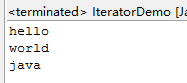## TreeSet类

• 概述
• 使用元素的自然顺序对元素进行排序
• 或者根据创建 set 时提供的 Comparator 进行排序
• 所以排序有两种方式
• 自然排序
• 比较器排序
• 具体取决于使用的构造方法。

public class TreeSetDemo {
public static void main(String[] args) {
// 创建集合对象
// 自然顺序进行排序
TreeSet<Integer> ts = new TreeSet<Integer>();

// 创建元素并添加
// 20,18,23,22,17,24,19,18,24

// 遍历
for (Integer i : ts) {
System.out.println(i);
}
}
}

• TreeSet是如何保证元素的排序和唯一性的
• 底层数据结构是红黑树(红黑树是一种自平衡的二叉树)

## 二叉树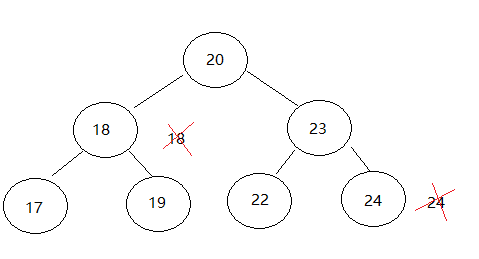## Comparator 排序

/*
* 需求：请按照姓名的长度排序
*/
public class TreeSetDemo {
public static void main(String[] args) {
// 创建集合对象
TreeSet<Student> ts = new TreeSet<Student>();

// 创建元素
Student s1 = new Student("朱婷", 22);
Student s2 = new Student("惠若琪", 22);
Student s3 = new Student("徐云丽", 21);
Student s4 = new Student("朱婷婷", 22);
Student s5 = new Student("郎平", 55);
Student s6 = new Student("林丹", 34);
Student s7 = new Student("李宗伟", 33);
Student s8 = new Student("阿杜", 23);

// 添加元素

// 遍历
for (Student s : ts) {
System.out.println(s.getName() + "---" + s.getAge());
}
}
}
/*
* 如果一个类的元素要想能够进行自然排序，就必须实现自然排序接口
*/
public class Student implements Comparable<Student> {
private String name;
private int age;

public Student() {
super();
}

public Student(String name, int age) {
super();
this.name = name;
this.age = age;
}

public String getName() {
return name;
}

public void setName(String name) {
this.name = name;
}

public int getAge() {
return age;
}

public void setAge(int age) {
this.age = age;
}

@Override
public int compareTo(Student s) {
// 主要条件 姓名的长度
int num = this.name.length() - s.name.length();
// 姓名的长度相同，不代表姓名的内容相同
int num2 = num == 0 ? this.name.compareTo(s.name) : num;
// 姓名的长度和内容相同，不代表年龄相同，所以还得继续判断年龄
int num3 = num2 == 0 ? this.age - s.age : num2;
return num3;
}
}

• TreeSet集合保证元素排序和唯一性的原理
• 唯一性：是根据比较的返回是否是0来决定。
• 排序：
• A:自然排序(元素具备比较性)
• 让元素所属的类实现自然排序接口 Comparable
• B:比较器排序(集合具备比较性)
• 让集合的构造方法接收一个比较器接口的子类对象 Comparator

• A:创建随机数对象
• B:创建一个HashSet集合
• C:判断集合的长度是不是小于10
• 是：就创建一个随机数添加
• 否：不理它
• D:遍历HashSet集合

public class HashSetDemo {
public static void main(String[] args) {
// 创建随机数对象
Random r = new Random();

// 创建一个Set集合
HashSet<Integer> ts = new HashSet<Integer>();

// 判断集合的长度是不是小于10
while (ts.size() < 10) {
int num = r.nextInt(20) + 1;
}

// 遍历Set集合
for (Integer i : ts) {
System.out.println(i);
}
}
}